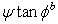The Rocscience International Conference 2021 Proceedings are now available. Read Now

# Unsaturated Shear Strength

The Unsaturated Shear Strength option in the Material Properties dialog allows you to account for shear strength induced by negative pore pressures (commonly referred to as matric suction) in the unsaturated zone above the water table. Unsaturated shear strength parameters are Phi_b and Air Entry Value.

Negative pore pressures can result from:

• interpolation of water pressure grid results (e.g. imported water pressure grid data obtained from in-situ measurements or finite element analysis)
• water surfaces (e.g. water table) (negative pore pressures are automatically computed above the water surface)

If negative pore pressures are calculated AND you define unsaturated shear strength parameters, then materials in the unsaturated zone above the water table will exhibit additional shear strength due to matric suction as described below.

Unsaturated Shear Strength Angle (Phi_b)

By specifying an Unsaturated Shear Strength Angle (Phi_b) for a material, the shear strength of the material in the unsaturated zone will be INCREASED by the amount:By default, the Unsaturated Shear Strength Angle = 0. This means that matric suction in the unsaturated zone, WILL NOT have any effect on the shear strength or safety factor. If you enter a non-zero value for Unsaturated Shear Strength, then slip surfaces which pass through a material in the unsaturated zone, will have INCREASED shear strength, and safety factor.

The Unsaturated Shear Strength Angle is usually not a well known quantity. To obtain an appreciation of its importance, a parametric study can be carried out, in which the Unsaturated Shear Strength Angle is varied between 0 and the Friction Angle of the material.

## Air Entry Value

Air Entry Value is defined in Fredlund and Rahardjo (1993) as the matric suction value that must be exceeded before air recedes into the soil pores. The Air Entry Value is also referred to as the "displacement pressure" in petroleum engineering or the "bubbling pressure" in ceramics engineering.

In terms of strength, it is the point at which the strength of the soil deviates from its saturated effective Mohr-Coulomb behavior. If matric suctions exceed the air entry value, the strength is assumed to follow a linear envelope with Phi_b as the friction angle. This gives a bilinear strength envelope where:

• c' and phi' are used with positive pore pressures and with negative pore pressures (matric suction) up to the air entry value
• Phi_b is used when negative pore pressure exceeds the air entry value.

The following figure (after Fredlund (2000) ) illustrates the effect of the air entry value. For matric suction below the air entry value, the saturated effective stress friction angle is used. For matric suction greater than the air entry value, the unsaturated shear strength angle, Phi_b is used.

Effect of air entry value (aev) on unsaturated shear strength envelopes## Negative Pore Pressure Cutoff

If you are using Unsaturated Shear Strength parameters for a material, the Negative Pore Pressure Cutoff option allows you to limit the contribution of negative pore pressure to the material shear strength. This option is in the Water Parameters tab of the Material Properties dialog.